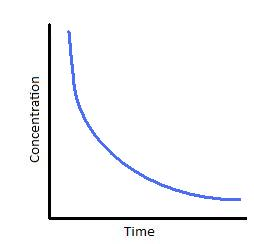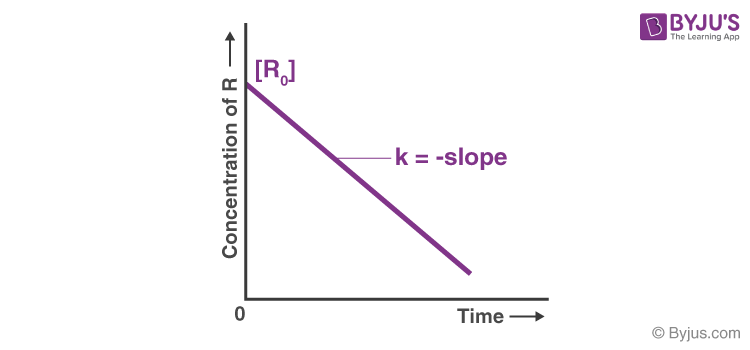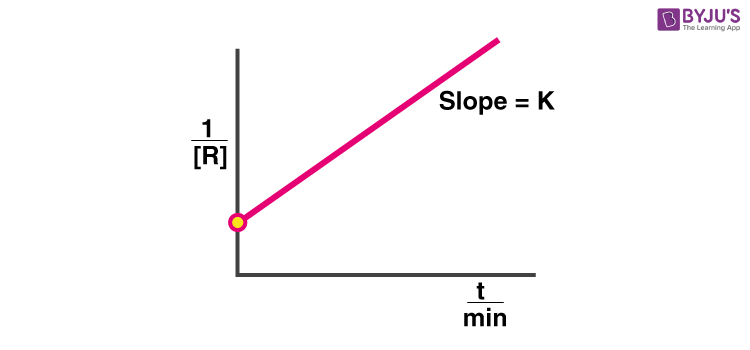Checkout JEE MAINS 2022 Question Paper Analysis : Checkout JEE MAINS 2022 Question Paper Analysis :

# Chemistry Practical Class 12 Reaction between Potassium Iodate (KIO3 ) and Sodium Sulphite (Na2 SO3) using the starch solution as indicator (clock reaction) Viva Questions with Answers

Q1. What is the concentration of sulphuric acid used in this experiment?

Q2. What is the concentration of sodium thiosulphate solution used in this experiment?

Q3. What is the percentage of potassium iodate solution used in this experiment?

Q4. What is the percentage of sodium sulphite solution used in this experiment?

Q5. What is the percentage of starch solution used in this experiment?

Q6. What is the colour of the starch-iodine complex?

Q7. Why can’t the molecularity of any reaction be equal to zero?

Answer: The molecularity of any reaction is the number of the reactant molecules or species colliding simultaneously in an elementary reaction. A minimum of one reactant is needed to initiate a chemical reaction. Therefore, the molecularity of the reaction cannot be zero.

Q8. What is the rate-determining step?

Answer: In complex reactions, the slowest step is the rate-determining step. It determines the overall reaction rate.

Q9. How will you plot a graph between the concentration and time of the first-order reaction?

Answer:Q10. On increasing the concentration of reactants, the reaction rate does not change. What can you say about the order of the reaction?

Answer: It is a zero-order reaction.

Q11. The reaction under examination is as follows :

S2O32- (aq) + 2H+ (aq) → H2O (l) + SO2 (g) + S(g)

Write the conditions under which the rate law expression for this reaction can be written in the following manner.

Rate of precipitation of sulphur = k [S2O32- ] [H+]2.

Answer: None of the reactants should be used in excess, and the reaction should be elementary.

Q12. Suppose the above rate law expression for the precipitation of sulphur holds good, then on doubling the concentration of S2O32- ion and H+ ion, by how many times will the rate of the reaction increase?

Answer: It will increase by eight times.

Q13. “For an exothermic reaction, the activation energy for the forward reaction is less than that for the backward reaction.” Is this statement true or false?

Answer: Yes, the above statement is true.

Q14. How will you plot a graph between the concentration and time of the zero-order reaction?

Answer:Q15. What is threshold energy?

Answer: Threshold energy is the minimum kinetic energy that all colliding molecules must have to make effective collisions between two reactant molecules.

Q16. What are the factors that affect the reaction rate?

Answer: Various factors can affect the chemical reaction rate.

• Temperature
• Concentration of the reactant
• Physical state
• Catalyst

Q17. Why are reactions with molecularity of more than three rare?

Answer: Reaction with a molecularity of more than three is rare because a simultaneous collision between more than three particles is rare.

Q18. How will you plot a graph between the concentration and time of the second-order reaction?

Answer:Q19. What is the law of mass action?

Answer: Law of Mass Action states that the rate of the chemical reaction is proportional to the product of the masses of the reacting substances, with each mass raised to a power equal to the coefficient that occurs in the chemical equation.

Q20. What is the effect of a catalyst on the activation energy and heat of the reaction?

Answer: A catalyst provides an alternate pathway by reducing the activation energy of the reaction. It does not affect the heat of the reaction.

Download Class 12 Chemistry Viva questions on the Reaction of Iodide ions with Hydrogen peroxide at room temperature using different concentrations of Iodide ions by clicking on the button below.